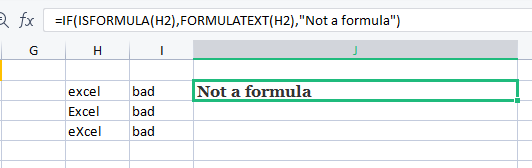# How to show formula as text in Excel

August 1, 2022
967 Views
0

A free Office suite fully compatible with Microsoft Office

When you enter formulas in cells in Microsoft Excel, the calculated results are often displayed. How will you handle it if you occasionally need to display merely the formula in a cell, such as =CONCATENATE(000, - 2)? There are various approaches to solving this issue:

# Show Formulas with Excel FORMULATEXT

The FORMULATEXT function can be applied to auditing and troubleshooting. For instance:

1. Display the formula of a cell

2. Determine how long the formula is.

3. Display the formula in a certain cell.

4. Display the formula or notice if there is no formula in the cell.

FORMULATEXT Syntax

FORMULATEXT(reference)

The following syntax applies to the FORMULATEXT function.

A single cell or a group of cells can be the reference.

# Show a cell's formula

The formula in a worksheet cell is shown as a text string via the FORMULATEXT function.

1. The formula input in cell I2 is displayed by the following formula, which is entered in cell J2.

2.   Additionally, you can refer to cells on different worksheets or workbooks.

3.   The formula will, however, provide a #N/A! error if another workbook is referenced but is not open.

4. The formula will appear in the upper left cell of the range of cells you referenced in the formula result if you do. The formula in cell B8 is returned in the following screenshot.

# Show formula in specific cell

Use FORMULATEXT and the INDIRECT function to build a troubleshooting and auditing tool. The formula in the designated cell can then be seen by entering a cell address in the referenced cell.

A cell address (B2) is entered in cell B4 in the picture below, and the FORMULATEXT result displays the formula in cell B2.

=FORMULATEXT(INDIRECT(B4))

# Show formula or message

An #N/A! error is produced if a cell without a formula is referenced by the FORMULATEXT function.

1. Instead of a formula, cell H2 in the picture below includes a text file called Excel. Cell J2 displays the outcome as a #N/A! mistake.

=FORMULATEXT(H2)

2. To determine whether a formula is present in the referred cell, use the ISFORMULA function (new in Excel 2013). Show the wording of the formula, if one exists. Display a message such as Not a formula if there is no formula.

Instead of a formula, cell H2 in the screenshot below has a text file called Excel. The outcome is Not a formula in cell J2.

#1.   Replicate Ste.

p 1 from the earlier procedure. In the Replace Command Box, type Equal (=) in the Find What box and press the Space key once & Equal (=) in the Replace With box. Select Find All.

2.   Next, select Replace All.

3.   Next, select Replace All.